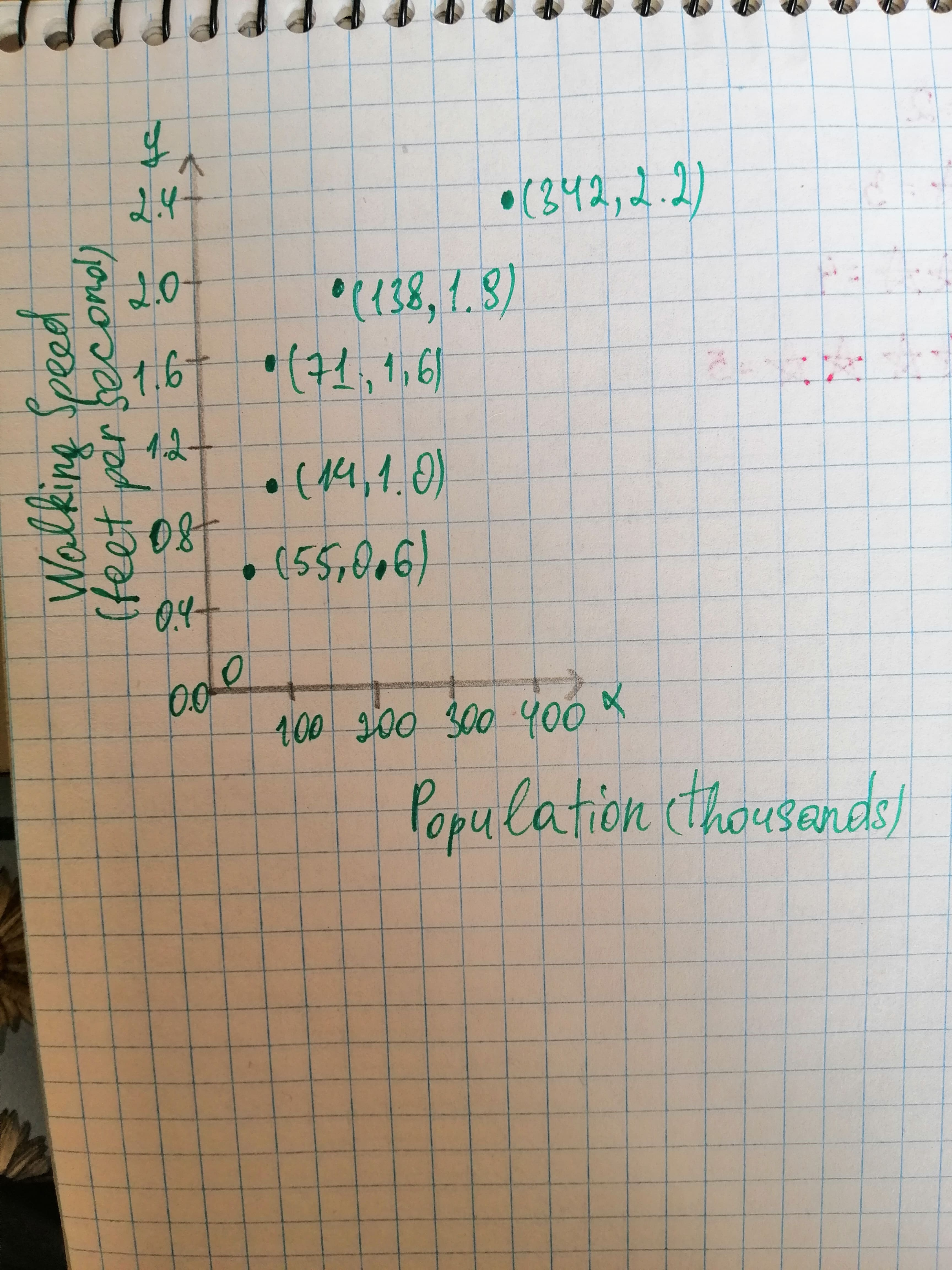Question# The table shows the population of various cities, in thousands, and the average walking speed, in feet per second, of a person living in the city

Modeling data distributions
ANSWEREDThe table shows the population of various cities, in thousands, and the average walking speed, in feet per second, of a person living in the city. $$\begin{array}{|c|c|} \hline Population\ (thousands) & Walking Speed\ (feet\ per\ second) \\ \hline 5.5 & 0.6 \\ \hline 14 & 1.0\\ \hline 71 & 1.6\\ \hline 138 & 1.9\\ \hline 342 & 2.2\\ \hline \end{array}$$Graph: Consider Walking speed as y-coordinates and population as x-coordinate and the table for this is given below: $$\begin{array}{|c|c|} \hline Population\ (thousands) & Walking Speed\ (feet\ per\ second) \\ \hline x-coordinates & y-coordinates\\ \hline 5.5 & 0.6 \\ \hline 14 & 1.0\\ \hline 71 & 1.6\\ \hline 138 & 1.9\\ \hline 342 & 2.2\\ \hline \end{array}$$
Use these points to plot the scatter plot. Thus, the scatter plot for the data is:Interpretation: The data in the scatter plot increase rapidly at first and then begin to level off a bit, the shape suggests that the logarithmic function is good choice for modeling the data.